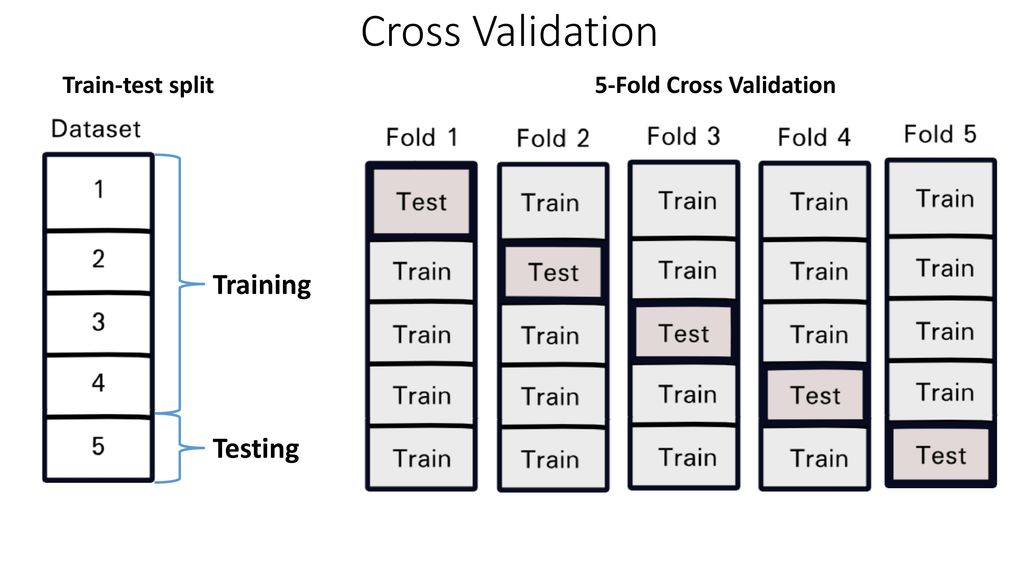# Catboost and Cross-Validation

You will learn how to use cross-validation and catboost.

In this notebook you can find an implementation of CatBoostClassifier and cross-validation for better measures of model performance!With this notebook, you will increase the stability of your models. So, we I will use K-Folds technique because its a popular and easy to understand. I will use 5 Folds.

## Plan:

1. Split dataset into 5 Folds.
2. Fit the model on 4 folds and validate using remaining fold.
3. Repeat this 5 times.
4. Inference our models on test data and submit them.

# Preparation¶

Installing `aicrowd-cli`

Login to `aicrowd-cli`

In [ ]:
```!pip install -q aicrowd-cli
!pip install -q catboost

!rm -rf data
!mkdir data
%aicrowd ds dl -c obstacle-prediction -o data
```
```     |████████████████████████████████| 43 kB 566 kB/s
|████████████████████████████████| 170 kB 7.1 MB/s
|████████████████████████████████| 54 kB 1.8 MB/s
|████████████████████████████████| 62 kB 481 kB/s
|████████████████████████████████| 211 kB 39.9 MB/s
|████████████████████████████████| 63 kB 1.1 MB/s
|████████████████████████████████| 51 kB 4.0 MB/s
ERROR: pip's dependency resolver does not currently take into account all the packages that are installed. This behaviour is the source of the following dependency conflicts.
google-colab 1.0.0 requires requests~=2.23.0, but you have requests 2.26.0 which is incompatible.
datascience 0.10.6 requires folium==0.2.1, but you have folium 0.8.3 which is incompatible.
|████████████████████████████████| 67.4 MB 65 kB/s
API Key valid
Saved API Key successfully!
```

# Importing Libraries¶

In [ ]:
```import pandas as pd
import numpy as np
from sklearn.model_selection import KFold
import os
import matplotlib.pyplot as plt
import seaborn as sns
from catboost import CatBoostClassifier
```

Reading the dataset and converting it to 1D array to train models

In [ ]:
```data = np.load("/content/data/data.npz", allow_pickle=True)

train_data = data["train"]
test_data = data['test']

X = np.array([sample.flatten() for sample in train_data[:, 0].tolist()])
y = np.array(train_data[:, 1].tolist())
```

# Training the Model¶

In [ ]:
```kf = KFold(n_splits=5, shuffle=True)
models = []

for i, (train_index, valid_index) in enumerate(kf.split(X)):
X_train, y_train = X[train_index], y[train_index]
X_valid, y_valid = X[valid_index], y[valid_index]

model = CatBoostClassifier(
iterations = 2,
depth = 1,
verbose = 10
)
model.fit(X_train, y_train, eval_set=(X_valid, y_valid))
models.append(model)
```
```Learning rate set to 0.5
0:	learn: 0.2479607	test: 0.2433617	best: 0.2433617 (0)	total: 28.2ms	remaining: 28.2ms
1:	learn: 0.1481542	test: 0.1546721	best: 0.1546721 (1)	total: 52.8ms	remaining: 0us

bestTest = 0.1546721212
bestIteration = 1

Learning rate set to 0.5
0:	learn: 0.2484560	test: 0.2436852	best: 0.2436852 (0)	total: 32.6ms	remaining: 32.6ms
1:	learn: 0.1344401	test: 0.1384810	best: 0.1384810 (1)	total: 57.8ms	remaining: 0us

bestTest = 0.1384810321
bestIteration = 1

Learning rate set to 0.5
0:	learn: 0.2317814	test: 0.2539978	best: 0.2539978 (0)	total: 25ms	remaining: 25ms
1:	learn: 0.1433883	test: 0.1635133	best: 0.1635133 (1)	total: 49.3ms	remaining: 0us

bestTest = 0.1635132789
bestIteration = 1

Learning rate set to 0.5
0:	learn: 0.2484638	test: 0.2416877	best: 0.2416877 (0)	total: 25ms	remaining: 25ms
1:	learn: 0.1477234	test: 0.1408042	best: 0.1408042 (1)	total: 49.7ms	remaining: 0us

bestTest = 0.1408041609
bestIteration = 1

Learning rate set to 0.5
0:	learn: 0.2465769	test: 0.2447656	best: 0.2447656 (0)	total: 24.8ms	remaining: 24.8ms
1:	learn: 0.1531736	test: 0.1421859	best: 0.1421859 (1)	total: 49.5ms	remaining: 0us

bestTest = 0.1421858874
bestIteration = 1

```

# Inference¶

In [ ]:
```# Converting each testing sample into 1D array
X_test = [sample.flatten() for sample in test_data.tolist()]

predictions = np.array([0. for i in range(len(X_test))])
for model in models:
preds = model.predict_proba(X_test)
predictions += np.array([pr for pr in preds])
predictions = [1 if pr > 0.5 else 0 for pr in predictions]
```
In [ ]:
```submission = pd.DataFrame({"label":predictions})
```
Out[ ]:
label
0 1
1 1
2 0
3 1
4 0
In [ ]:
```# Saving the pandas dataframe
!rm -rf assets
!mkdir assets
submission.to_csv(os.path.join("assets", "submission.csv"), index=False)
```

# Submitting our Predictions¶

Note : Please save the notebook before submitting it (Ctrl + S)

In [ ]:
```!aicrowd notebook submit -c obstacle-prediction -a assets --no-verify
```
2746konstantin_diachkov
About 1 year ago

Hi, why didn’t you divide the sum of probabilities by the number of models (5 in this case)?dmitriy_kutsenko
About 1 year ago

Yes you’re right, but I think that in this contest we have a very large data dimension and model can based just on small part of them, so just 1 model can find obstackle and set prediction to 1.